Determination of earthquake magnitudes using duration of high-frequency energy radiation and maximum displacement amplitudes: application to the April 1, 2007 Solomon Islands Earthquake

Tatsuhiko Hara

International Institute of Seismology and Earthquake Engineering, Building Research Institute

Recently, we developed a new method to determine earthquake magnitudes using the following formula (Hara, 2007):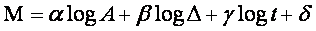where M is an earthquake magnitude,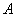is the maximum displacement during high-frequency energy radiation from the arrival time of a P-wave,is the epicentral distance,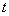is duration of high-frequency energy radiation. The duration of high-frequency energy radiation can be estimated by band-pass filtering of first arriving P-waves (Lomax, 2005; Park et al., 2005; Ni et al., 2005; Hara, 2007).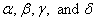are 0.79, 0.83, 0.69, and 6.47, respectively (the units of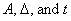were m, km, and s, respectively).

We applied this method to the April 1, 2007 Solomon Islands Earthquake (the origin time: 20:39:56UTC; the location 8.453°N, 156.957°E after USGS). Figure 1 shows an example of measurements of high-frequency energy radiation. The estimated duration is 92.5 sec, which is consistent with the centroid time shift 44.7 s of the Global CMT solution. The estimated magnitude using the above formula is 8.1, which agrees well with Mw 8.1 of the Global CMT solution.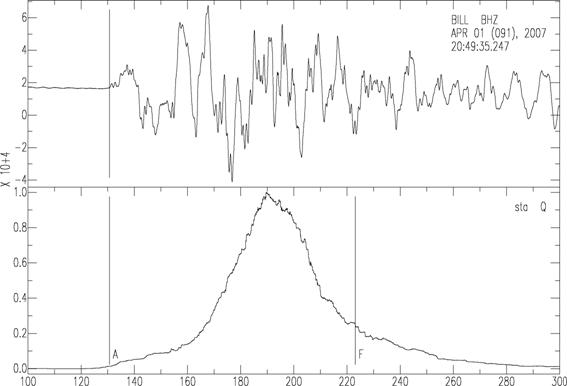Fig. 1. An example of measurements of high frequency energy radiation. The upper and lower traces are an observed seismogram and its smoothed time series of squares of band-pass (2-4 Hz) filtered seismogram (normalized by the maximum value), respectively. “A” and “F” in the lower trace denote the arrival of P-wave and estimated end of high frequency energy radiation, respectively.

References

Hara, T., Measurement of duration of high-frequency energy radiation and its application to determination of magnitudes of large shallow earthquakes, accepted for publication in Earth Planets Space, 2007.

Lomax, A., Rapid estimation of rupture extent for large earthquakes: Application to the 2004, M9 Sumatra-Andaman mega-thrust, Geophys. Res. Lett., 32, L10314, doi:10.1029/2005GL022437, 2005.

Ni, S., H. Kanamori, and D. Helmberger, Energy radiation from the Sumatra earthquake, Nature, 434, 582, 2005.

Park, J., K. Anderson, R. Aster, R. Butler, T. Lay, and D. Simpson, Global seismographic network records the great Sumatra-Andaman earthquake, Eos Trans. AGU, 86(6), 57, 2005.

Last Updated: 2007/04/03